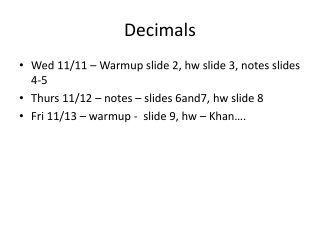DownloadDownload PresentationDecimals

# Decimals

Télécharger la présentation## Decimals

- - - - - - - - - - - - - - - - - - - - - - - - - - - E N D - - - - - - - - - - - - - - - - - - - - - - - - - - -
##### Presentation Transcript

1. Decimals • Wed 11/11 – Warmup slide 2, hw slide 3, notes slides 4-5 • Thurs 11/12 – notes – slides 6and7, hw slide 8 • Fri 11/13 – warmup - slide 9, hw – Khan….

2. Draw the number lines. Place the decimals on the number line and then use < or > to make the expression true. Warm-Up Ordering Decimals

3. Ordering Decimals Use <, >, or equal sign to make each expression true.

4. Notes: Ordering Decimals Decimals use place values. The places are located to the right of decimal point. Each place value is ten times the place value to its right.

5. Read the entire number to the right of the decimal point as if it is represented as a whole number, and then give the place value of the digit farthest to the right. Decimals and whole numbers may be combined to give decimals for numbers greater than 1. Example: 14.35 is read “fourteen and thirty-five hundredths.” Adding zeros to the right of a decimal does not change the number represented.

6. Notes: Adding and Subtracting Decimals Rules • Write the given numbers one above the other with the decimal points in line. • Add zeros to the right of the decimal to get the same number of decimal places and then add or subtract as if the numbers were whole numbers. • Place a decimal point in the number for the sum or difference in position under the decimal points in the given numbers Example 6.47 +340.8 13.94 – 7.693 would be written as 6.47 would be written as: 13.940 + 34 0.8 0- 7.693 420.793 6.247

7. Estimating Decimals To find an estimated sum or difference, first round to the highest place value of the smallest number, then compute. Example Add 8.574 + 81.03 + 59.432 First find the sum. 8.574 81.030 + 59.432 149.036 2. To check your answer, round all addends to the nearest unit. 8.574 9 81.030 81 59.432 59 3. Find the sum of the rounded addends. 9 + 81 + 59=149 The actual answer is in close agreement with the estimate. The actual answer is probably correct.

8. HW Adding & Subtracting Decimals Estimate the answer and the Add or Subtract. 9.86 2. 15.7 3. 9.87 4. 15.72 + 1.12+ 74.2- 3.52- 9.64 9.1 – 5.628 6. -0.1125 – 1.0297 7. 8 -3.095 8. 0.48 + 2.9015 9. 16.3 – 9.8602 10. -18.5 + 6.29

9. Warm-Up Adding & Subtracting Decimals 121.56 + 40.131 2. -79.92 + 16.213 -561.92 – 41.83 4. 108.6 – (-18.03)